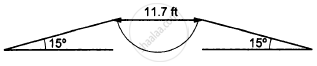Department of Pre-University Education, KarnatakaPUC Karnataka Science Class 11
Advertisement Remove all ads

# Figure (3−E8) Shows a 11.7 Ft Wide Ditch with the Approach Roads at an Angle of 15° with Horizontal. with What Minimum Speed Should a Motorbike Be Moving on Road So that It Safely Crosses the Ditch? - Physics

Short Note

In the following figure shows a 11.7 ft wide ditch with the approach roads at an angle of 15° with the horizontal. With what minimum speed should a motorbike be moving on the road so that it safely crosses the ditch?

Assume that the length of the bike is 5 ft, and it leaves the road when the front part runs out of the approach road.Advertisement Remove all ads

#### Solution

Given:
Width of the ditch = 11.7 ft
Length of the bike = 5 ft
The approach road makes an angle of 15˚ (α) with the horizontal.
Total horizontal range that should be covered by the biker to cross the ditch safely, R = 11.7 + 5 = 16.7 ft
Acceleration due to gravity, a = g = 9.8 m/s = 32.2 ft/s2
We know that the horizontal range is given by

$R = \frac{u^2 \sin2\alpha}{g}$

By putting respective values, we get:

$u^2 = \frac{Rg}{\sin2\alpha} = \frac{16 . 7 \times 32 . 2}{\sin30^\circ}$

$\Rightarrow u \approx 32 ft/s$

Therefore, the minimum speed with which the motorbike should be moving is 32 ft/s.

Is there an error in this question or solution?
Advertisement Remove all ads

#### APPEARS IN

HC Verma Class 11, 12 Concepts of Physics 1
Chapter 3 Rest and Motion: Kinematics
Q 36 | Page 52
Advertisement Remove all ads
Advertisement Remove all ads
Share
Notifications

View all notifications

Forgot password?